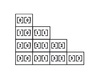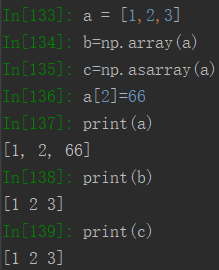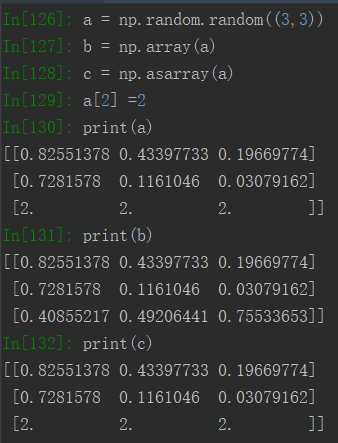array 订阅

Array

• ## np.asarray()和np.array()区别

千次阅读 多人点赞 2019-08-21 21:22:06
如果输入是list，np.array() 和np.asarray()都可以理解为“深拷贝”,也就是修改a不影响b,c 如果输入是array, np.array()理解为“深拷贝”（修改a，不影响b的值）和np.asarray()理解为"浅拷贝"(修改a，影响c的值...


如果输入是list，np.array() 和np.asarray()都可以理解为“深拷贝”,也就是修改a不影响b,c如果输入是array,  np.array()理解为“深拷贝”（修改a，不影响b的值）和np.asarray()理解为"浅拷贝"(修改a，影响c的值）展开全文• 这篇文章主要介绍了JS Array.slice 截取数组的实现方法,因为我们需要控制一下长度，需要的朋友可以参考下 slice定义和用法 slice() 方法可从已有的数组中返回选定的元素。 语法 arrayObject.slice(start,end) ...


这篇文章主要介绍了JS Array.slice 截取数组的实现方法,因为我们需要控制一下长度，需要的朋友可以参考下

slice定义和用法

slice() 方法可从已有的数组中返回选定的元素。

语法
arrayObject.slice(start,end)

参数
描述
start
必需。规定从何处开始选取。如果是负数，那么它规定从数组尾部开始算起的位置。也就是说，-1 指最后一个元素，-2 指倒数第二个元素，以此类推。
end
可选。规定从何处结束选取。该参数是数组片断结束处的数组下标。如果没有指定该参数，那么切分的数组包含从 start 到数组结束的所有元素。如果这个参数是负数，那么它规定的是从数组尾部开始算起的元素。

返回值

返回一个新的数组，包含从 start 到 end （不包括该元素）的 arrayObject 中的元素。

说明

请注意，该方法并不会修改数组，而是返回一个子数组。如果想删除数组中的一段元素，应该使用方法 Array.splice()。

提示和注释

注释：您可使用负值从数组的尾部选取元素。
注释：如果 end 未被规定，那么 slice() 方法会选取从 start 到数组结尾的所有元素。

实例

例子 1

在本例中，我们将创建一个新数组，然后显示从其中选取的元素：

<script type="text/javascript">

var arr = new Array(3)
arr = "George"
arr = "John"
arr = "Thomas"

document.write(arr + "<br />")
document.write(arr.slice(1) + "<br />")
document.write(arr)

</script>

输出：
George,John,Thomas
John,Thomas
George,John,Thomas

例子 2

在本例中，我们将创建一个新数组，然后显示从其中选取的元素：

<script type="text/javascript">

var arr = new Array(6)
arr = "George"
arr = "John"
arr = "Thomas"
arr = "James"
arr = "Martin"

document.write(arr + "<br />")
document.write(arr.slice(2,4) + "<br />")
document.write(arr)

</script>

输出：
Thomas,James

核心代码：

<script type="text/javascript">
//JS Array.slice 截取数组
//在JavaScript中，Array对象的slice(start[,end])方法返回数组从下标[start,end)的部分（不包含下标为end的元素）如果没有指定end参数，则从start开始到数组结尾的部分，slice()方法不改变原数组，如果要删除数组的一部分，可以使用splice()方法。
//参数：
//（1）start:开始截取的数组下标，如果start是负数，表明从数组尾部开始计算。
//（2）end:结束截取的数组下标，如果end是负数，表明从数组尾部开始计算。
//例1：

var arr = [1,2,3,4,5,6,7,8,9];
// [0,1,2,3,4,5,6,7,8]
// [-10,-9,-8,-7,-6,-5,-4,-3,-2,-1]

document.writeln(arr.slice(5)); // 输出：6,7,8,9
document.writeln(arr.slice(-5)); // 输出：5,6,7,8,9
document.writeln(arr.slice(0,3)); // 输出：1,2,3
document.writeln(arr.slice(1,2)); // 输出：2
document.writeln(arr.slice(3,-2)); // 输出：4,5,6,7
document.writeln(arr.slice(1,9999)); // 输出：2,3,4,5,6,7,8,9
//==================================================================================================
//JS Array.splice(start,delete_count,value,...) 插入、删除、替换数组
//参数：
//（1）start:开始插入和（或）删除的数组元素的下标。
//（2）delete_count:结束截取的数组下标，如果end是负数，表明从数组尾部开始计算。
//（3）value,...：要插入数组的元素。
//返回：如果从数组中删除了元素，则返回的是被删除的元素的数组
//
//例1：
document.write("<hr>");
//
var arr = [1,2,3,4,5,6,7,8,9];
document.writeln("arr=" + arr); // 输出：arr=1,2,3,4,5,6,7,8,9
document.writeln("arr.splice(5)=" + arr.splice(5)); // 输出：arr.splice(5)=6,7,8,9
document.writeln("arr=" + arr); // 输出：arr=1,2,3,4,5
document.write("<br>");
//
var arr = [1,2,3,4,5,6,7,8,9];
document.writeln("arr=" + arr); // 输出：arr=1,2,3,4,5,6,7,8,9
document.writeln("arr.splice(5,1,99,100)=" + arr.splice(5,1,99,100)); // 输出：arr.splice(5,1,99,100)=6
document.writeln("arr=" + arr); // 输出：arr=1,2,3,4,5,99,100,7,8,9
document.write("<br>");
</script>

ok了，其实就是使用array.slice(0,20);截取前20个。

展开全文• 1、np.mat() np.mat()函数用于将输入解释为矩阵。 np.mat(data, dtype=None) 不同于np.matrix，如果输入本身就已经是matrix或ndarray ，则np.asmatrix不会复制输入，而是...data：array_like。输入数据。 dtype：...
欢迎关注WX公众号：【程序员管小亮】
np.mat()函数用于将输入解释为矩阵。
np.matrix()函数用于从类数组对象或数据字符串返回矩阵。
np.array()函数用于创建一个数组。
1、np.mat()
np.mat(data, dtype=None)

不同于np.matrix，如果输入本身就已经是matrix或ndarray ，则np.asmatrix不会复制输入，而是仅仅创建了一个新的引用。
相当于np.matrix(data, copy=False)。
参数：

data：array_like。输入数据。

dtype：数据类型。输出矩阵的数据类型。

返回：

mat：矩阵。数据被解释为矩阵。

例子1：
>>> x = np.array([[1, 2], [3, 4]])
>>> m = np.asmatrix(x)
>>> x[0,0] = 5
>>> m
matrix([[5, 2],
[3, 4]])

2、np.matrix()
class numpy.matrix(data, dtype=None, copy=True)


注意
不再建议使用此类，即使对于线性代数也是如此。而是使用常规数组。该课程将来可能会被删除。

矩阵是一种专用的二维阵列，通过操作保留其二维特性。
它有一些特殊的运算符，如* （矩阵乘法）和 **（矩阵幂）。
参数：

data ： array_like或string。如果data是字符串，则将其解释为以逗号或空格分隔列的矩阵，以及分隔行的分号。

dtype ： 数据类型。输出矩阵的数据类型。

copy ： 布尔。如果data已经是ndarray，则此标志确定是复制数据（默认值）还是构造视图。

例子2：
>>> a = np.matrix('1 2; 3 4')
>>> a
matrix([[1, 2],
[3, 4]])

>>> np.matrix([[1, 2], [3, 4]])
matrix([[1, 2],
[3, 4]])

3、np.array()
numpy.array(object,
dtype=None,
copy=True,
order='K',
subok=False,
ndmin=0)

参数：

object ： array_like
数组，公开数组接口的任何对象，__array__方法返回数组的对象，或任何（嵌套）序列。

dtype ： 数据类型，可选
数组所需的数据类型。如果没有给出，那么类型将被确定为保持序列中的对象所需的最小类型。此参数只能用于“upcast”数组。对于向下转换，请使用.astype（t）方法。

copy ： bool，可选
如果为true（默认值），则复制对象。否则，只有当__array__返回副本，obj是嵌套序列，或者需要副本来满足任何其他要求（dtype，顺序等）时，才会进行复制。

order ： {‘K’，‘A’，‘C’，‘F’}，可选
指定阵列的内存布局。如果object不是数组，则新创建的数组将按C顺序排列（行主要），除非指定了’F’，在这种情况下，它将采用Fortran顺序（专业列）。如果object是一个数组，则以下成立。

order
no copy
copy=True

‘K’
unchanged
F & C order preserved, otherwise most similar order

‘A’
unchanged
F order if input is F and not C, otherwise C order

‘C’
C order
C order

‘F’
F order
F order

当copy=False出于其他原因而复制时，结果copy=True与对A的一些例外情况相同。默认顺序为“K”。

subok ： bool，可选。如果为True，则子类将被传递，否则返回的数组将被强制为基类数组（默认）。

ndmin ： int，可选。指定结果数组应具有的最小维数。为满足此要求，将根据需要预先设置形状。

返回：

out ： ndarray。满足指定要求的数组对象。

例子3：
>>>
>>> np.array([1, 2, 3])
array([1, 2, 3])

上溯造型：
>>>
>>> np.array([1, 2, 3.0])
array([ 1.,  2.,  3.])

不止一个方面：
>>>
>>> np.array([[1, 2], [3, 4]])
array([[1, 2],
[3, 4]])

最小尺寸2：
>>>
>>> np.array([1, 2, 3], ndmin=2)
array([[1, 2, 3]])

提供的类型：
>>>
>>> np.array([1, 2, 3], dtype=complex)
array([ 1.+0.j,  2.+0.j,  3.+0.j])

由多个元素组成的数据类型：
>>>
>>> x = np.array([(1,2),(3,4)],dtype=[('a','<i4'),('b','<i4')])
>>> x['a']
array([1, 3])
py
从子类创建数组：
py
>>>
>>> np.array(np.mat('1 2; 3 4'))
array([[1, 2],
[3, 4]])
>>>
>>> np.array(np.mat('1 2; 3 4'), subok=True)
matrix([[1, 2],
[3, 4]])

python课程推荐。展开全文• 为什么要用numpy  Python中提供了list容器，可以当作数组使用。但列表中的元素可以是任何对象，... Python虽然也提供了array模块，但其只支持一维数组，不支持多维数组(在TensorFlow里面偏向于矩阵理解)，也...


为什么要用numpy

Python中提供了list容器，可以当作数组使用。但列表中的元素可以是任何对象，因此列表中保存的是对象的指针，这样一来，为了保存一个简单的列表[1,2,3]。就需要三个指针和三个整数对象。对于数值运算来说，这种结构显然不够高效。
Python虽然也提供了array模块，但其只支持一维数组，不支持多维数组(在TensorFlow里面偏向于矩阵理解)，也没有各种运算函数。因而不适合数值运算。
NumPy的出现弥补了这些不足。

（——摘自张若愚的《Python科学计算》）

import numpy as np

数组创建

## 常规创建方法
a = np.array([2,3,4])
b = np.array([2.0,3.0,4.0])
c = np.array([[1.0,2.0],[3.0,4.0]])
d = np.array([[1,2],[3,4]],dtype=complex) # 指定数据类型
print a, a.dtype
print b, b.dtype
print c, c.dtype
print d, d.dtype

[2 3 4] int32
[ 2.  3.  4.] float64
[[ 1.  2.]
[ 3.  4.]] float64
[[ 1.+0.j  2.+0.j]
[ 3.+0.j  4.+0.j]] complex128

数组的常用函数
print np.arange(0,7,1,dtype=np.int16) # 0为起点，间隔为1时可缺省(引起歧义下不可缺省)
print np.ones((2,3,4),dtype=np.int16) # 2页，3行，4列，全1，指定数据类型
print np.zeros((2,3,4)) # 2页，3行，4列，全0
print np.empty((2,3)) #值取决于内存
print np.arange(0,10,2) # 起点为0，不超过10，步长为2
print np.linspace(-1,2,5) # 起点为-1，终点为2，取5个点
print np.random.randint(0,3,(2,3)) # 大于等于0，小于3，2行3列的随机整数

[0 1 2 3 4 5 6]
[[[1 1 1 1]
[1 1 1 1]
[1 1 1 1]]

[[1 1 1 1]
[1 1 1 1]
[1 1 1 1]]]
[[[ 0.  0.  0.  0.]
[ 0.  0.  0.  0.]
[ 0.  0.  0.  0.]]

[[ 0.  0.  0.  0.]
[ 0.  0.  0.  0.]
[ 0.  0.  0.  0.]]]
[[  1.39069238e-309   1.39069238e-309   1.39069238e-309]
[  1.39069238e-309   1.39069238e-309   1.39069238e-309]]
[0 2 4 6 8]
[-1.   -0.25  0.5   1.25  2.  ]
[[1 0 1]
[0 1 0]]

类型转换
print float(1)
print int(1.0)
print bool(2)
print float(True)

1.0
1
True
1.0

数组输出

从左到右，从上向下
一维数组打印成行，二维数组打印成矩阵，三维数组打印成矩阵列表

print np.arange(1,6,2)
print np.arange(12).reshape(3,4) # 可以改变输出形状
print np.arange(24).reshape(2,3,4)# 2页，3行，4页

[1 3 5]
[[ 0  1  2  3]
[ 4  5  6  7]
[ 8  9 10 11]]
[[[ 0  1  2  3]
[ 4  5  6  7]
[ 8  9 10 11]]

[[12 13 14 15]
[16 17 18 19]
[20 21 22 23]]]

基本运算

## 元素级运算
a = np.array([1,2,3,4])
b = np.arange(4)
print a, b
print a-b
print a*b
print a**2
print 2*np.sin(a)
print a>2
print np.exp(a) # 指数

[1 2 3 4] [0 1 2 3]
[1 1 1 1]
[ 0  2  6 12]
[ 1  4  9 16]
[ 1.68294197  1.81859485  0.28224002 -1.51360499]
[False False  True  True]
[  2.71828183   7.3890561   20.08553692  54.59815003]

## 矩阵运算（二维数组）
a = np.array([[1,2],[3,4]]) # 2行2列
b = np.arange(6).reshape((2,-1)) # 2行3列
print a,b
print a.dot(b) # 2行3列

[[1 2]
[3 4]] [[0 1 2]
[3 4 5]]
[[ 6  9 12]
[12 19 26]]

## 非数组运算，调用方法
a = np.random.randint(0,5,(2,3))
print a
print a.sum(),a.sum(axis=1),a.sum(0) # axis用于指定运算轴（默认全部，可指定0或1）
print a.min(),a.max(axis=1),a.mean(axis=1) # axis = 0: 按列计算，axis = 1: 按行计算
print a.cumsum(1) # 按行计算累积和

[[2 3 3]
[0 2 1]]
11 [8 3] [2 5 4]
0 [3 2] [ 2.66666667  1.        ]
[[2 5 8]
[0 2 3]]

索引，切片，迭代

## 一维数组
a = np.arange(0,10,1)**2
print a
print a,a,a[-1],a[-2] # 索引从0开始，-1表示最后一个索引
print a[2:5],a[-5:-1] # 包括起点，不包括终点
a[-1] = 100; print a # 赋值
a[1:4]=100; print a # 批量赋值
a[:6:2] = -100; print a # 从开始到第6个索引，每隔一个元素（步长=2）赋值
print a[: :-1];print a # 将a逆序输出，a本身未发生改变
b = [np.sqrt(np.abs(i)) for i in a]; print b # 通过遍历赋值

[ 0  1  4  9 16 25 36 49 64 81]
0 4 81 64
[ 4  9 16] [25 36 49 64]
[  0   1   4   9  16  25  36  49  64 100]
[  0 100 100 100  16  25  36  49  64 100]
[-100  100 -100  100 -100   25   36   49   64  100]
[ 100   64   49   36   25 -100  100 -100  100 -100]
[-100  100 -100  100 -100   25   36   49   64  100]
[10.0, 10.0, 10.0, 10.0, 10.0, 5.0, 6.0, 7.0, 8.0, 10.0]

## 多维数组
a = np.arange(0,20).reshape((4,5))
print a, a[2,3], a[:,1], a[1:4,2], a[1:3,:]
print a[-1] # 相当于a[-1,:],即索引少于轴数时，确实的索引默认为整个切片

b = np.arange(0,24).reshape((2,3,4))
print b,b # 相当于b[1,:,:] 和b[1,...]
print '-------------------'
for row in a:
print row # 遍历以第一个轴为基础

[[ 0  1  2  3  4]
[ 5  6  7  8  9]
[10 11 12 13 14]
[15 16 17 18 19]] 13 [ 1  6 11 16] [ 7 12 17] [[ 5  6  7  8  9]
[10 11 12 13 14]]
[15 16 17 18 19]
[[[ 0  1  2  3]
[ 4  5  6  7]
[ 8  9 10 11]]

[[12 13 14 15]
[16 17 18 19]
[20 21 22 23]]]

[[12 13 14 15]
[16 17 18 19]
[20 21 22 23]]
-------------------
[0 1 2 3 4]
[5 6 7 8 9]
[10 11 12 13 14]
[15 16 17 18 19]

形状操作

a = np.floor(10*np.random.random((3,4)))
print a, a.shape #输出a的形状
print a.ravel() # 输出平坦化后的a（a本身不改变）
a.shape = (6,2); print a # 改变a的形状
print a.transpose() # 输出a的转置

[[ 0.  4.  3.  2.]
[ 1.  1.  3.  3.]
[ 4.  4.  6.  5.]] (3, 4)
[ 0.  4.  3.  2.  1.  1.  3.  3.  4.  4.  6.  5.]
[[ 0.  4.]
[ 3.  2.]
[ 1.  1.]
[ 3.  3.]
[ 4.  4.]
[ 6.  5.]]
[[ 0.  3.  1.  3.  4.  6.]
[ 4.  2.  1.  3.  4.  5.]]

## 补充：reshape和resize
a = np.array([[1,2,3],[4,5,6]])
b = a
a.reshape((3,2))# 不改变数组本身的形状
print a
b.resize((3,2))# 改变数组本身形状
print b

[[1 2 3]
[4 5 6]]
[[1 2]
[3 4]
[5 6]]

---------------------

在numpy模块中，我们经常会使用resize 和 reshape,在具体使用中，通常是使用resize改变数组的尺寸大小，使用reshape用来增加数组的维度。

1.resize

之前看到别人的博客说，resize没有返回值，其实这取决于你如何使用resize,resize有两种使用方式，一种是没有返回值的，直接对原始的数据进行修改，还有一种用法是有返回值的，所以不会修改原有的数组值。

1.1有返回值，不对原始数据进行修改

import numpy as np
X=np.array([[1,2,3,4],
[5,6,7,8],
[9,10,11,12]])

X_new=np.resize(X,(3,3)) # do not change the original X
print("X:\n",X)  #original X
print("X_new:\n",X_new) # new X

>>
X:
[[ 1  2  3  4]
[ 5  6  7  8]
[ 9 10 11 12]]
X_new:
[[1 2 3]
[4 5 6]
[7 8 9]]

1.2 无返回值，直接修改原始数组的大小

import numpy as np
X=np.array([[1,2,3,4],
[5,6,7,8],
[9,10,11,12]])

X_2=X.resize((3,3))  #change the original X ,and do not return a value
print("X:\n",X)  # change the original X
print("X_2:\n",X_2) # return None

X:
[[1 2 3]
[4 5 6]
[7 8 9]]
X_2:
None

2.reshape

给数组一个新的形状而不改变其数据

import numpy as np
X=np.array([1,2,3,4,5,6,7,8])

X_2=X.reshape((2,4)) #retuen a 2*4 2-dim array
X_3=X.reshape((2,2,2)) # retuen a 2*2*2 3-dim array

print("X:\n",X)
print("X_2:\n",X_2)
print("X_3:\n",X_3)

>>
X:
[1 2 3 4 5 6 7 8]
X_2:
[[1 2 3 4]
[5 6 7 8]]
X_3:
[[[1 2]
[3 4]]

[[5 6]
[7 8]]]
---------------------
作者：墨晓白
原文：https://blog.csdn.net/qq_24193303/article/details/80965274

展开全文• python中找出numpy array数组的最值及其索引 在list列表中，max(list)可以得到list的最大值，list.index(max(list))可以得到最大值对应的索引 但在numpy中的array没有index方法，取而代之的是where，其又是list...numpy
• return (sizeof(array) / sizeof(array)); } int sort(int A[],int x) { for(int i=0;i<(sizeof(A)/sizeof(A));i++) { if(A[i]==x) { return i; break; } } } int main() { int A={0,...
• python实现两个三维数组的拼接 python中仅仅依靠调用numpy来实现三维数组的拼接没有对应的函数，但是遇到这样一个需求：每次循环生成一个矩阵，需要把每次循环的矩阵叠加成三维矩阵，例如： ...python
• numpy还是很强大的，这里把一些矩阵基本操作做一些整理，方便大家，也方便我自己码代码的时候查找。 有句话对于我这个初学者来说觉得还是挺符合的，翻书看视频浏览教程贴啊什么的，会发现很多知识点，一开始并不用...python numpy 函数 数组 矩阵
• int getArrayLen(T& array) //使用模板定义一个函数getArrayLen,该函数将返回数组array的长度 { return (sizeof(array) / sizeof(array)); } void ranking(int a[], int b[]){ int N = getArrayLen(a); cout ...函数值调用 模板
• 成功解决ValueError: Expected 2D array, got 1D array instead 目录 解决问题 解决思路 解决方法 解决问题 ValueError: Expected 2D array, got 1D array instead ...
• 成功解决ValueError: Expected 2D array, got 1D array instead: Reshape your data either using array.reshap 目录 解决问题 解决思路 解决方法 解决问题 ValueError: Expected 2D array, got 1D ...
• Array 和 ArrayList 有何区别？ Array 即数组，声明方式可以如下： int[] array = new int; int array [] = new int; int[] array = {1, 2, 3}; int[] array = new int[]{1, 2, 3}; 定义一个 Array 时...
• 错误截图 错误分析 这个错误的意思是说：无效的命名数据:“数据”类型检查失败。期望数组，得到对象，那么我们这个时候很明白了，是类型不对，但是是哪一行的呢？打开错误信息下面的详情，这个时候找到后缀是自己...vue element
• JS array 数组详解数组的声明方法arrayObj = new Array();//创建一个数组。 var arr1 = new Array(); arrayObj = new Array([size])//创建一个数组并指定长度，注意不是上限，是长度。 var a = new Array(5); ...javascript 数组操作
• array_map语法格式： array_map(function($v){ //TODO },$array); array_walk语法格式 array_walk($array, function($v, $k){ //TODO }); array_filter语法格式 array_filter($array, function($v)... • 一、背景 &...网上百度一下array_map，然后发现了array_walk。这两个函数在某些情境下有异曲同工之妙，然后区别还是很大的。 二、关于array_map 1、文档 &amp;nbsp;&amp;nbsp;&amp;php • array_map 对数组中每一个元素进行处理 // 初始化一组数据$data = [1, 2, 3, 4, 5, 6, 7, 8, 9, 10]; // 对数组中的每个元素 + 5 array_map(function ($item){ echo$item + 5 . ' '; }, \$data); echo '&lt;...
• Array.of方法用于将一组值，转换为数组。Array.of总是返回参数值组成的数组。如果没有参数，就返回一个空数组。Array.of基本上可以用来替代Array()或new Array()，并且不存在由于参数不同而导致的重载。它的行为非常...es6 扩展 javascript
• 当我们需要1000万个浮点数的时候，数组（array）的效率要比列表（list）要高得多，因为数组在背后存的并不是float对象，而是数字的机器翻译，也就是字节表述。这一点和c语言中的数组一样。 再比如说，如果需要频繁对...
• ## numpy: np.asarray 函数

万次阅读 2017-12-15 11:42:16
np.asarray(a, dtype=None, order=None) 将结构数据转化为ndarray。Parameters: a : array_like dtype : data-type, optional order : {‘C’, ‘F’}, optional Returns: out : ndarray 与 np.array 的不同np.as...numpy
• ## numpy中array和asarray的区别

万次阅读 多人点赞 2017-02-22 14:45:06
array和asarray都可以将结构数据转化为ndarray，但是主要区别就是当数据源是ndarray时，array仍然会copy出一个副本，占用新的内存，但asarray不会。 举例说明： import numpy as np #example 1: data1=[[1,1,1],...python numpy
• ## Scala之Array的方法

万次阅读 多人点赞 2018-08-05 11:06:14
Scala：Array（集合、序列） Scala开篇（目录） 数组是一种可变的、可索引的数据集合。在Scala中用Array[T]的形式来表示Java中的数组形式 T[]。 val numbers = Array(1, 2, 3, 4) //声明一个数组对象 val first =...
• 一、Image.fromarray的作用：实现array到image的转换 二、PIL中的Image和numpy中的数组array相互转换： 1. PIL image转换成array 2. array转换成image 一、Image.fromarray的作用： 简而言之，就是实现array...
• string_agg，array_agg 这两个函数的功能大同小异，只不过合并数据的类型不同。 https://www.postgresql.org/docs/9.6/static/functions-aggregate.htmlarray_agg(expression) 把表达式变成一个数组 一般配合 array...postgresql 数据 函数
• String s; char [] arr = s.toCharArray();//将String 转array String.valueOf(arr);//将array转String： https://www.runoob.com/java/number-valueof.html
• 1，创建一个字符串数组修改其中的索引值并打印 /** * Created by Administrator on 2018/1/28. */ public class DynamicArrayTest { public static final void main(String...... Class clazz =
• 成功解决ValueError: setting an array element with a sequence. 目录 解决问题 解决方法 解决问题 ValueError: setting an array element with a sequence. 解决方法 值错误：用序列设置...
• let array1:Array&amp;lt;number&amp;gt;; let array2:number[]; 二、数组初始化 let array1:Array&amp;lt;number&amp;gt; = new Array&amp;lt;number&amp;gt;(); let ...typescript 数组...